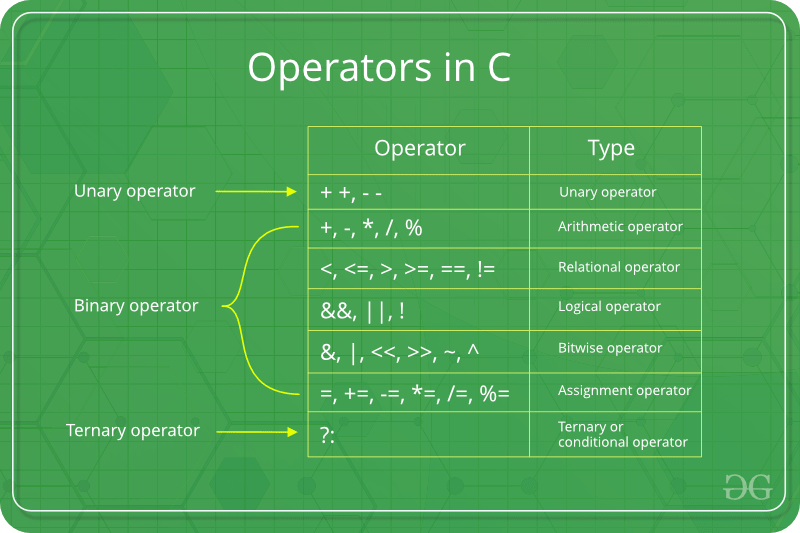Skip to content
Related Articles
Bitwise Operators in C/C++
• Difficulty Level : Medium
• Last Updated : 04 Nov, 2019

In C, the following 6 operators are bitwise operators (work at bit-level)1. The & (bitwise AND) in C or C++ takes two numbers as operands and does AND on every bit of two numbers. The result of AND is 1 only if both bits are 1.
2. The | (bitwise OR) in C or C++ takes two numbers as operands and does OR on every bit of two numbers. The result of OR is 1 if any of the two bits is 1.
3. The ^ (bitwise XOR) in C or C++ takes two numbers as operands and does XOR on every bit of two numbers. The result of XOR is 1 if the two bits are different.
4. The << (left shift) in C or C++ takes two numbers, left shifts the bits of the first operand, the second operand decides the number of places to shift.
5. The >> (right shift) in C or C++ takes two numbers, right shifts the bits of the first operand, the second operand decides the number of places to shift.
6. The ~ (bitwise NOT) in C or C++ takes one number and inverts all bits of it

Example:

 `// C Program to demonstrate use of bitwise operators``#include ``int` `main()``{``    ``// a = 5(00000101), b = 9(00001001)``    ``unsigned ``char` `a = 5, b = 9;`` ` `    ``// The result is 00000001``    ``printf``(``"a = %d, b = %d\n"``, a, b);``    ``printf``(``"a&b = %d\n"``, a & b);`` ` `    ``// The result is 00001101``    ``printf``(``"a|b = %d\n"``, a | b);`` ` `    ``// The result is 00001100``    ``printf``(``"a^b = %d\n"``, a ^ b);`` ` `    ``// The result is 11111010``    ``printf``(``"~a = %d\n"``, a = ~a);`` ` `    ``// The result is 00010010``    ``printf``(``"b<<1 = %d\n"``, b << 1);`` ` `    ``// The result is 00000100``    ``printf``(``"b>>1 = %d\n"``, b >> 1);`` ` `    ``return` `0;``}`
Output:
```a = 5, b = 9
a&b = 1
a|b = 13
a^b = 12
~a = 250
b<<1 = 18
b>>1 = 4
```

Interesting facts about bitwise operators

1. The left shift and right shift operators should not be used for negative numbers. If any of the operands is a negative number, it results in undefined behaviour. For example results of both -1 << 1 and 1 << -1 is undefined. Also, if the number is shifted more than the size of integer, the behaviour is undefined. For example, 1 << 33 is undefined if integers are stored using 32 bits. See this for more details.
2. The bitwise XOR operator is the most useful operator from technical interview perspective. It is used in many problems. A simple example could be “Given a set of numbers where all elements occur even number of times except one number, find the odd occurring number” This problem can be efficiently solved by just doing XOR of all numbers.
 `#include `` ` `// Function to return the only odd``// occurring element``int` `findOdd(``int` `arr[], ``int` `n)``{``    ``int` `res = 0, i;``    ``for` `(i = 0; i < n; i++)``        ``res ^= arr[i];``    ``return` `res;``}`` ` `// Driver Method``int` `main(``void``)``{``    ``int` `arr[] = { 12, 12, 14, 90, 14, 14, 14 };``    ``int` `n = ``sizeof``(arr) / ``sizeof``(arr);``    ``printf``(``"The odd occurring element is %d "``,``           ``findOdd(arr, n));``    ``return` `0;``}`
Output:
```The odd occurring element is 90
```

The following are many other interesting problems using XOR operator.

.

3. The bitwise operators should not be used in place of logical operators. The result of logical operators (&&, || and !) is either 0 or 1, but bitwise operators return an integer value. Also, the logical operators consider any non-zero operand as 1. For example, consider the following program, the results of & and && are different for same operands.
 `#include `` ` `int` `main()``{``    ``int` `x = 2, y = 5;``    ``(x & y) ? ``printf``(``"True "``) : ``printf``(``"False "``);``    ``(x && y) ? ``printf``(``"True "``) : ``printf``(``"False "``);``    ``return` `0;``}`
Output:
```False True
```
4. The left-shift and right-shift operators are equivalent to multiplication and division by 2 respectively. As mentioned in point 1, it works only if numbers are positive.
 `#include `` ` `int` `main()``{``    ``int` `x = 19;``    ``printf``(``"x << 1 = %d\n"``, x << 1);``    ``printf``(``"x >> 1 = %d\n"``, x >> 1);``    ``return` `0;``}`
Output:
```x << 1 = 38
x >> 1 = 9
```
5. The & operator can be used to quickly check if a number is odd or even. The value of expression (x & 1) would be non-zero only if x is odd, otherwise the value would be zero.
 `#include `` ` `int` `main()``{``    ``int` `x = 19;``    ``(x & 1) ? ``printf``(``"Odd"``) : ``printf``(``"Even"``);``    ``return` `0;``}`
Output:
```Odd
```
6. The ~ operator should be used carefully. The result of ~ operator on a small number can be a big number if the result is stored in an unsigned variable. And the result may be a negative number if the result is stored in a signed variable (assuming that the negative numbers are stored in 2’s complement form where the leftmost bit is the sign bit)
 `// Note that the output of the following``// program is compiler dependent``#include `` ` `int` `main()``{``    ``unsigned ``int` `x = 1;``    ``printf``(``"Signed Result %d \n"``, ~x);``    ``printf``(``"Unsigned Result %ud \n"``, ~x);``    ``return` `0;``}`
Output:
```Signed Result -2
Unsigned Result 4294967294d
```

Important Links:

Please write comments if you find anything incorrect, or you want to share more information about the topic discussed above

Attention reader! Don’t stop learning now. Get hold of all the important DSA concepts with the DSA Self Paced Course at a student-friendly price and become industry ready.  To complete your preparation from learning a language to DS Algo and many more,  please refer Complete Interview Preparation Course.

In case you wish to attend live classes with industry experts, please refer DSA Live Classes

My Personal Notes arrow_drop_up